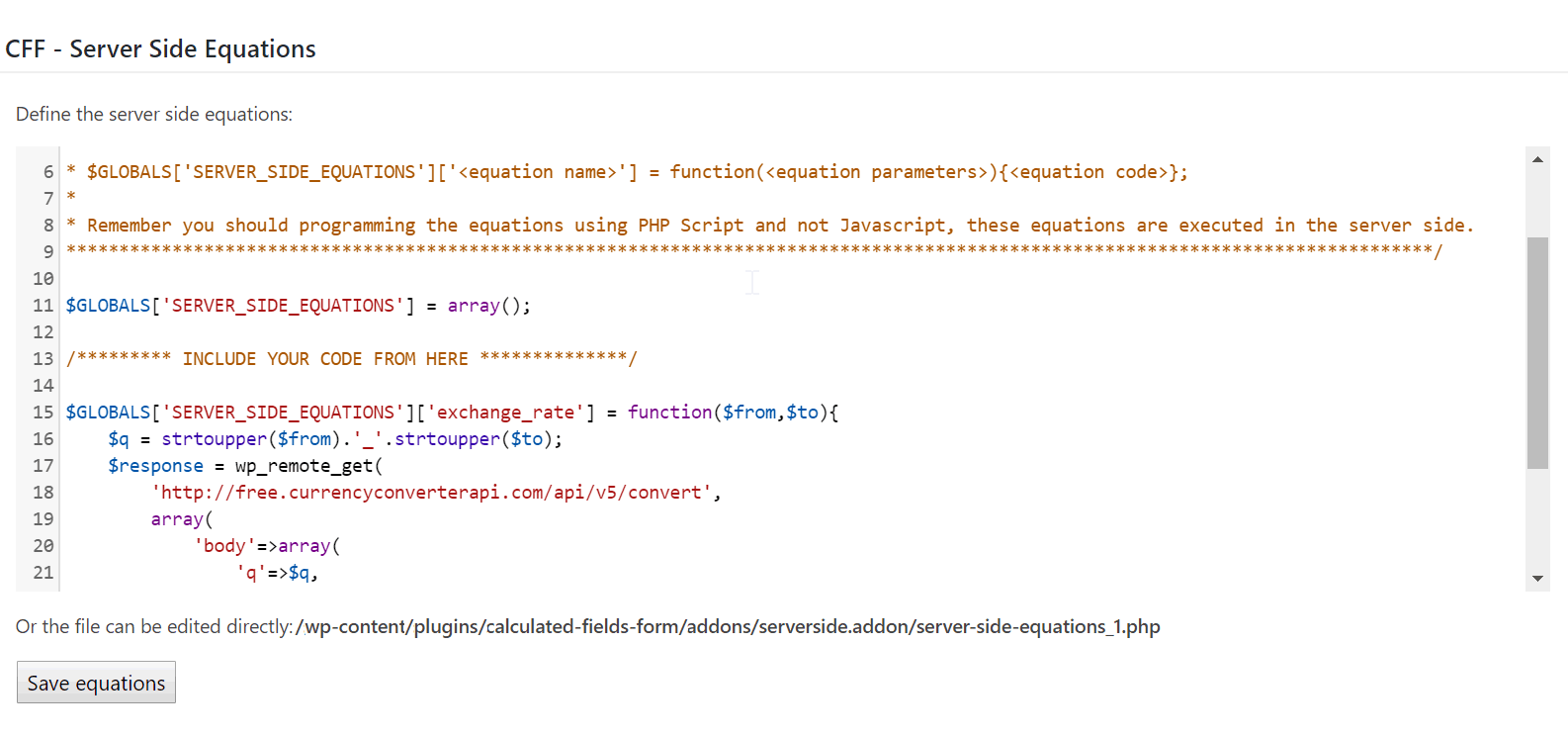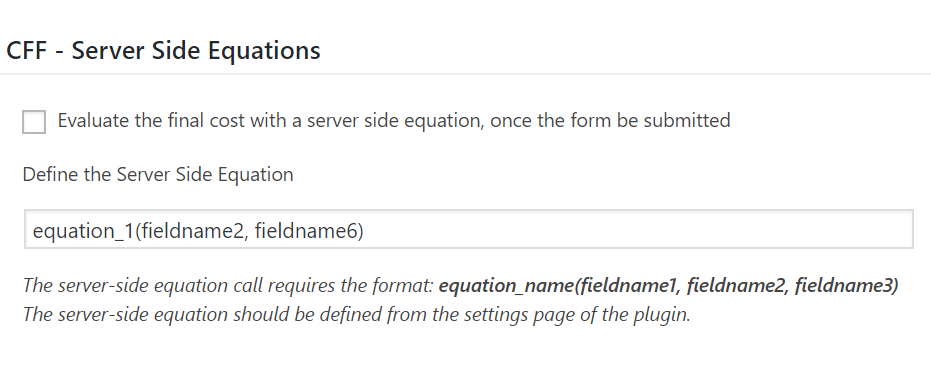# Calculated Fields Form Blog

Tips and cases of use for a successful WordPress website with calculated forms.

Blog / Server Side Equations

## Server Side Equations

The server side equations are the alternative to use server side code or the server resources in the equations, access to external APIs or services, and hide the business logic.

With the Calculated Fields Form plugin is very easy create formulas to:

• calculate the price of products at runtime in e-commerces like WooCommerce,
• calculate the body mass index of people for some Health type of business,
• calculate the distance-time and price of travels for transportation companies,
• calculate the lodging cost based on date ranges and seasons,

And more.

The possibilities are infinite...

The formulas are implemented in javascript and evaluated in the client side (the browser).

But, what to do if you need to use the server resources? Or in the case of needing the potential of PHP or the WordPress functions (for example, for sending emails with a summary of the users' progress)? Or What to do if the project requires the integration with external services, but hiding the accesses to the services and the integration's logic?

The answer to all these questions are the Server Side Equations (or Server Side Formulas).

The Server Side Equations are possible using the "Server Side Equations" add-on, distributed with the Developer and Platinum versions of the plugin. These equations are implemented with server side code and called from the calculated fields in the form.

##### How to use the Server Side Equations.

The first step is activate the add-on: go to the settings page of the plugin through the WordPress menu option: "Settings > Calculated Fields Form", tick the checkbox: "CFF-Server Side Equations", and press the "Activate/Deactivate addons" button.

Once the add-on is enabled, the plugin displays a code editor for implementing the server side equations. By the way thank you very much to the WordPress team for integrate an code editor with syntax highlight in WordPress.I guess that would be more beneficial for everyone if I describe the process with a real case of use.

Describing the problem:

I've created a form to calculate the price of a service in Euros (EUR), but many of my users are americans, and I need to display the service's price in both currencies: Euros and United State Dollar (USD). So, would be necessary integrate the form with an Exchange Rate service, to get the exchange rate in real time.

After analyze the different alternatives I've decided to use the free service:

http://free.currencyconverterapi.com/api/v5/convert

The service's URL should be called passing to it two parameters: q=EUR_USD for the currencies involved in the conversion, and compat=y to get the result in compact mode.

The first step would be implement the server side equation, as I said above, the equations are entered through the code editor: "Define the server side equations" in the settings page of the plugin, available after activate the add-on.

A server side equation has the structure:

\$GLOBALS['SERVERSIDEEQUATIONS'][<equation name>] = function(<the parameters separated by comma>) { <the code of the equation> };

For this project, the equation can be implemented as follows:

``````\$GLOBALS['SERVER_SIDE_EQUATIONS']['exchange_rate'] = function(\$from,\$to){
\$q = strtoupper(\$from).'_'.strtoupper(\$to);
\$response = wp_remote_get(
'http://free.currencyconverterapi.com/api/v5/convert',
array(
'body'=>array(
'q'=>\$q,
'compact'=>'y'
)
)
);
\$body = json_decode(wp_remote_retrieve_body(\$response));
return \$body->\$q->val;
};
``````

I've named my equation as: exchange_rate. It requires two parameters, \$from and \$to for the currency codes. The code of the equation can seem complex, but summarizing, it uses the wpremoteget function of WordPress for accessing to the service's URL, whose result is a json object that I'm converting into a PHP object with the PHP function json_decode, and very important, the equation includes a "return" instruction for returning the exchange rate.

Once the server side equation is defined, it is time to use it from the form. The plugin includes the "SERVER_SIDE" operation for calling the server side equations. The first parameter of SERVER_SIDE operation is the name of the server side equation, the other parameters of the operation would be the parameters of the server side equation (two additional parameters in this case for the \$from and \$to parameters).

Therefore, going back to the hypothetical example and assumptions, if the fieldname1 field in the form calculates the service's price in Euros, to get this price in USD, I need only to insert another calculated field with the following equation:

``````fieldname1*SERVER_SIDE('exchange_rate', 'EUR', 'USD')
``````

Now, the form is able to calculate the service's price in Euros and Dollars.

##### Pros and Cons of Server Side Equations:

Pros:

• The server side equations are reusable. They are implemented only one time but can be called from multiple forms.
• The equations' codes are stored in the server, hiding the business logic.
• Allows to use server side code and the WordPress functions.

Cons:

• In the server side's equations cannot be used the operations defined by the plugin because they are implemented with javascript, and the server side equations must be implemented with PHP.
• The evaluation of the equations will have a little delay, because would be needed a call to the web server.
##### Calculating the final price with a server side equation

The Server Side Equations add-on includes a new section in the form's settings to allow calculate the final price through a server side equation, after the form be submitted.To calculated the final price with a server side equation:

• Tick the checkbox: "Evaluate the final cost with a server side equation, once the form be submitted"

• Enter the server side equation call with the format:

equation_name(fieldname1, fieldname2, fieldname3)